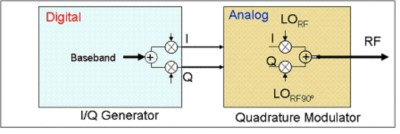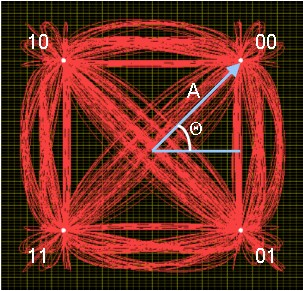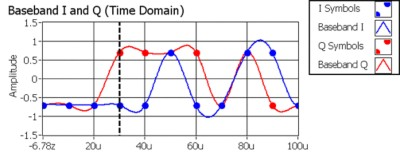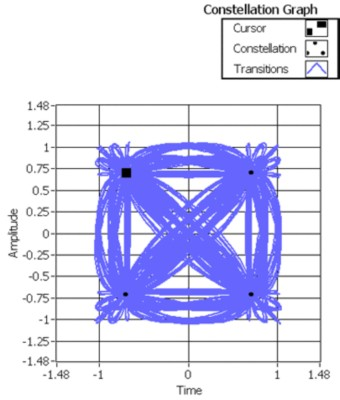## Überblick

This tutorial is part of the National Instruments Measurement Fundamentals series. Each tutorial in this series teaches you a specific topic of common measurement applications by explaining the theory and giving practical examples. This tutorial covers an introduction to RF, wireless, and high-frequency signals and systems.

For the complete list of tutorials, return to the NI Measurement Fundamentals main page, or for more RF tutorials, refer to the NI RF Fundamentals main subpage. For more information about National Instruments RF products, visit www.ni.com/rf.

A variety of communication protocols implement quadrature amplitude modulation (QAM). Current protocols such as 802.11b wireless Ethernet (Wi-Fi) and digital video broadcast (DVB), for example, both utilize 64-QAM modulation. In addition, emerging wireless technologies such as Worldwide Interoperability for Microwave Access (WiMAX), 802.11n, and HSDPA/HSUPA (a new cellular data standard) will implement QAM as well. Thus, understanding QAM is important because of its widespread use in current and emerging technologies.

QAM involves sending digital information by periodically adjusting the phase and amplitude of a sinusoidal electromagnetic wave. Each combination of phase and amplitude is called a symbol and represents a digital bitstream. This tutorial first covers the hardware implementation required to constantly adjust the phase and amplitude of a carrier wave. The tutorial also discusses the binary value associated with each symbol.

## Hardware Implementation

Quadrature amplitude modulation (QAM) requires changing the phase and amplitude of a carrier sine wave. One of the easiest ways to implement QAM with hardware is to generate and mix two sine waves that are 90 degrees out of phase with one another. Adjusting only the amplitude of either signal can affect the phase and amplitude of the resulting mixed signal.

These two carrier waves represent the in-phase (I) and quadrature-phase (Q) components of our signal. Individually each of these signals can be represented as:

I = A cos(φ) and Q = A sin(φ).

Note that the I and Q components are represented as cosine and sine because the two signals are 90 degrees out of phase with one another. Using the two identities above and the following trigonometric identity

cos(α + β) = cos(α)cos(β) – sin(α)sin(β),

rewrite a carrier wave A cos(2πfct + φ) as

A cos(2πfct + φ) = I cos(2πfct) – Q sin(2πfct).

As the equation above illustrates, the resulting identity is a periodic signal whose phase can be adjusted by changing the amplitude of I and Q. Thus, it is possible to perform digital modulation on a carrier signal by adjusting the amplitude of the two mixed signals.

Figure 1 shows a block diagram of the hardware required to generate the intermediate frequency (IF) signal. The “Quadrature Modulator” block shows how the I and Q signals are mixed with the local oscillator (LO) signal before being mixed together. The two LOs are exactly 90 degrees out of phase with one another.Figure 1. Hardware Used to Generate the IF Signal

The next section discusses exactly how the I and Q components are used to represent actual digital data. To do this, the following section details the relationship between the QAM symbol map and the actual real-world signal.

## QAM Symbol Map

As stated previously, QAM involves sending digital information by periodically adjusting the phase and amplitude of a sinusoidal electromagnetic wave. Four-QAM uses four combinations of phase and amplitude. Moreover, each combination is assigned a 2-bit digital pattern. For example, suppose you want to generate the bitstream (1,0,0,1,1,1). Because each symbol has a unique 2-bit digital pattern, these bits are grouped in two’s so that they can be mapped to the corresponding symbols. In our example, the original bitstream (1,0,0,1,1,1) is grouped into the three symbols (10,01,11).

In the following figure, 4-QAM consists of four unique combinations of phase and amplitude. These combinations—called symbols—are shown as the white dots on the constellation plot in Figure 2. The red lines represent the phase and amplitude transitions from one symbol to another. Labeled on the constellation plot is the digital bit pattern that each symbol represents. Thus, a digital bit pattern can be sent over a carrier signal by generating unique combinations of phase and amplitude.Figure 2. 4-QAM Symbol Map

How do these digital bit patterns correspond to I/Q data? Figure 3 shows both the I/Q data (top) and the 2-bit digital pattern (in a constellation plot, bottom) relating to 4-QAM. The black dashed line in the top graphic corresponds to the black square marker in the bottom graphic, so you can follow the phase and amplitude changes as the I/Q parameters also change.Figure 3. (top) 4-QAM Baseband I/Q Data (bottom) 4-QAM Constellation Plot

The constellation plot in Figure 2 shows the symbol map of 4-QAM with each possible phase (Θ) and amplitude (A) of a carrier signal in polar coordinate form. Notice how in the bottom graphic of Figure 3 the square marker passes over each symbol at least once.

While it is possible to send up to two bits per symbol when using 4-QAM modulation, it is also possible to send data at even higher rates by increasing the number of symbols in our symbol map. By convention, the number of symbols in a symbol map is called the symbol map “M” and is considered the “M-ary” of the modulation scheme. In other words, 4-QAM has an M-ary of four and 256-QAM has an M-ary of 256. Moreover, the number of bits that can be represented by a symbol has a logarithmic relationship to the M-ary. For example, we know that two bits can be represented by each symbol in 4-QAM. While this makes sense intuitively, it is defined by the equation bits per symbol = log2 (M).

Using this equation, each symbol in 256-QAM can be used to represent an 8-bit digital pattern (log2 (256) = 8). Because the M-ary of a QAM modulation scheme affects the number of bits per symbol, the M-ary has a significant affect on the actual data transmission rate.

## Conclusions

QAM is an important modulation scheme because of its widespread adoption in current technologies. Moreover, this scheme can be implemented in LabVIEW with the use of the NI Modulation Toolkit. This toolkit, in conjunction with the vector signal generator and vector signal analyzer, implements QAM for signals in the real world.

For the complete list of tutorials, return to the NI Measurement Fundamentals main page, or for more RF tutorials, refer to the NI RF Fundamentals main subpage. For more information about National Instruments RF products, visit www.ni.com/rf.

## Related Products

NI PXIe-5663 6.6 GHz RF Vector Signal Analyzer
The National Instruments PXIe-5663 is a modular 6.6 GHz RF vector signal analyzer with 50 MHz of instantaneous bandwidth optimized for automated test.

NI PXIe-5673 6.6 GHz RF Vector Signal Generator
The National Instruments PXIe-5673 is a 4-slot 6.6 GHz RF vector signal generator that delivers signal generation from 85 MHz to 6.6 GHz, 100 MHz of instantaneous bandwidth, and up to 512 MB of memory.

NI PXI-5660 2.7 GHz RF Vector Signal Analyzer
The National Instruments PXI-5660 is a modular 2.7 GHz RF vector signal analyzer with 20 MHz of instantaneous bandwidth optimized for automated test.

NI PXI-5671 2.7 GHz RF Vector Signal Generator
The National Instruments PXI-5671 module is a 3-slot RF vector signal generator that delivers signal generation from 250 kHz to 2.7 GHz, 20 MHz of instantaneous bandwidth, and up to 512 MB of memory.

NI PXI-5652 6.6 GHz RF and Microwave Signal Generator
The National Instruments PXI-5652 6.6 GHz RF and microwave signal generator is continuous-wave with modulation capability. It is excellent for setting up stimulus response applications with RF signal analyzers.

NI RF Switches
The National Instruments RF switch modules are ideal for expanding the channel count or increasing the flexibility of systems with signal bandwidths greater than 10 MHz to bandwidths as high as 26.5 GHz.

NI LabVIEW
National Instruments LabVIEW is an industry-leading graphical software tool for designing test, measurement, and automation systems.

## References

1. Haykin, Simon. Communications Systems. 3rd ed. Canada: John Wiley & Sons, Inc., 1994.
2. Lathi, B. P. Modern Digital and Analog Communication Systems. 3rd ed. USA: Oxford University Press, 1998.

## Conclusion

For the complete list of tutorials, return to the NI Measurement Fundamentals main page, or for more RF tutorials, refer to the NI RF Fundamentals main subpage. For more information about National Instruments RF products, visit www.ni.com/rf.